WOW !! MUCH LOVE ! SO WORLD PEACE !
Fond bitcoin pour l'amélioration du site: 1memzGeKS7CB3ECNkzSn2qHwxU6NZoJ8o
Dogecoin (tips/pourboires): DCLoo9Dd4qECqpMLurdgGnaoqbftj16NvpRechercher sur le site:

# Current effect of endogenous and exogenous uncertainty on the exchange rate in the next two years (ZAR/USD)

( Télécharger le fichier original )
University of Johannesburg - Master's articles 2010

# II.1 Exchange rate and fundamentals

a) Flexi-price Monetary model

The model concentrates on the current account and assumes prices are flexible and output is exogenous, determine by the supply side of the economy. It's relies on the PPP condition and a stable demand for money function. The logarithm of the demand for money may be assumed to depend on the log of real income, Y, the price level, P, and the level of the interest rate, r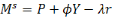(Domestic equilibrium) (2.3)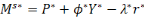(Foreign equilibrium) (2.4)

Where ? is a coefficient parameter of real income and ë the coefficient of the interest rate. In this model the domestic r is exogenous because of the assumption of perfect capital mobility and a zero expected change in the exchange rate. The equilibrium in traded exchange market ensues when prices in a common currency are equalised; by using the PPP condition: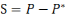(2.5)

b) Dornbusch overshooting model

In 1976, Dornbusch model begin with a description of the main behavioural assumption. The uncovered interest parity relationship expresses the conditions for equilibrium. Foreign exchange speculators investing abroad expect a return of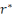+u%, wherethe foreign interest rate and u is is the expected appreciation of foreign currency (depreciation in domestic currency).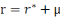(2.6)

the expectation about the exchange rate are assumed to be regressive; if the actual rate lies below the long run equilibrium rate, s, therefore the expectation of actual rate to rise towards the long-run(Keith, p293);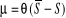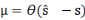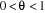(2.7)

The rational expectation in this formula allows having a correct expectation: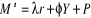(Equation in the money market)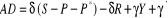(Good market)

Where the first term represents the impact of real exchange rate on net trade volumes, the second (-är) is the invest schedule, the third term (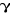Y) the consumption function and expenditure effects on imports and the final term (') exogenous demand factors such as government expenditure.

c) Frankel real interest differential model

In 1979 Jeffrey Frankel provides a model for analysing the impact of change in the interest rate on the exchange rate and he refers to this as «the real interest differential model». This model assumes uncovered arbitrage but modifies the Dornbusch expectation equation for the exchange rate by adding a term reflecting relative expected secular inflation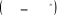the expectation equation is(Keith p295):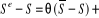And an uncovered interest parity yield is: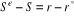The term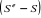is the expected rate of depreciation which depends upon the deviation of the exchange rate from its equilibrium value. If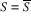, the expected rate of depreciation is given by the expected inflation differential.